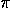# Historical Overview of PiThe ancient Babylonians knew of the existence of- the ratio of the circumference to the diameter of any circle. The constant they obtained, 3.125, made use of their knowledge that the perimeter of a regular hexagon inscribed in a circle equals six times the radius of the circle. By using this perimeter of the inscribed hexagon as a lower bound for the circumference of the circle, they were able to come up with their remarkably close approximation forcirca 2000 B.C. [2, p.21].

During the fith century B.C., Hippias of Elis discovered the quadratrix, a curve which could be used to determinethrough a geometric construction. Hippias' quadratrix, could be used to "square the circle" although not in a manner acceptable to the ancient Greek (or even modern) geometers. "Squaring the circle" was the problem posed by the ancient Greeks in which a square was to be constructed with area equal to that of a given circle [2, p.40].

In the third century B.C., Archimedes of Syracuse, widely accepted as the greatest scientific mind of antiquity, found a method wherebycould be determined to any degree of accuracy desired. This Archimedean method for computinginscribed and circumscribed regular polygons in and around the circle. His conception of the use of upper and lower bounds reached the threshold of the differential calculus. The Archimedean value ofwas 3 10/71 << 3 1/7 .

In Fifth Century A.D. China, the mathematician Tsu Chung-Chi established that 3.1415926 << 3.1415927 an accuracy that was not attained in Europe until the 16th Century [2, p.29]. The Chinese, like Archimedes could calculateto any degree of accuracy desired.

Leonardo da Vinci (in the 16th Century) and a 17th Century Japanese document found the approximate area of a cicle by rearrangement. This method breaks the circle into equal sectors and arranges them into an approximate parallelogram. This method is not sophisticated, so it is suspected that a form of this method was used by ancient peoples to find the area of a circle [2, p.19].

The discovery of infinite series representations forby such mathematicians as Gregory, Leibniz and Euler in the 17th and 18th century made it possible to calculate ¼ to scores of digits relatively easily. By 1666 Newton had found a way to calculate, using his binomial theorem, to 16 decimal places using only 22 terms of an infinite series expansion .

In 1882, the German mathematician, F. Lindemann proved the numberand its square root to be transcendental numbers, ones which cannot be roots of polynomial equations with integer coefficients [1, p.23]. Lindemann's discovery settled the circle squaring problem posed by the Greeks showing it to be impossible using straight edge and compass and the rules of the ancient Greek geometers.

Nowdays it is easy to get more digits ofthan are really useful. What was once difficult to approximate to three or four digits is now a trivial computer computation to hundreds of thousands of digits. However, computing many digits ofis a good test of a computer's speed and accuracy. Included is the approximate value ofto 100,000 digits.

Somthing new always turns up with. In 1995 an interesting relationship was discovered betweenand the Fibonacci numbers. An exact numerical representation ofcan be made using an arbitrary number of Fibonacci numbers . This relationship can also be demonstrated geometrically.http://www.geom.umn.edu/~huberty/math5337/groupe/overview.html